# Optimization Methods¶

Until now, you've always used Gradient Descent to update the parameters and minimize the cost. In this notebook, you will learn more advanced optimization methods that can speed up learning and perhaps even get you to a better final value for the cost function. Having a good optimization algorithm can be the difference between waiting days vs. just a few hours to get a good result.

Gradient descent goes "downhill" on a cost function $J$. Think of it as trying to do this:**Figure 1** : **Minimizing the cost is like finding the lowest point in a hilly landscape**
At each step of the training, you update your parameters following a certain direction to try to get to the lowest possible point.

Notations: As usual, $\frac{\partial J}{\partial a } =$ da for any variable a.

To get started, run the following code to import the libraries you will need.

In :
import numpy as np
import matplotlib.pyplot as plt
import scipy.io
import math
import sklearn
import sklearn.datasets

from opt_utils import compute_cost, predict, predict_dec, plot_decision_boundary, load_dataset
from testCases import *

%matplotlib inline
plt.rcParams['figure.figsize'] = (7.0, 4.0) # set default size of plots
plt.rcParams['image.interpolation'] = 'nearest'
plt.rcParams['image.cmap'] = 'gray'


A simple optimization method in machine learning is gradient descent (GD). When you take gradient steps with respect to all $m$ examples on each step, it is also called Batch Gradient Descent.

Warm-up exercise: Implement the gradient descent update rule. The gradient descent rule is, for $l = 1, ..., L$: $$W^{[l]} = W^{[l]} - \alpha \text{ } dW^{[l]} \tag{1}$$ $$b^{[l]} = b^{[l]} - \alpha \text{ } db^{[l]} \tag{2}$$

where L is the number of layers and $\alpha$ is the learning rate. All parameters should be stored in the parameters dictionary. Note that the iterator l starts at 0 in the for loop while the first parameters are $W^{}$ and $b^{}$. You need to shift l to l+1 when coding.

In :
# GRADED FUNCTION: update_parameters_with_gd

"""
Update parameters using one step of gradient descent

Arguments:
parameters -- python dictionary containing your parameters to be updated:
parameters['W' + str(l)] = Wl
parameters['b' + str(l)] = bl
learning_rate -- the learning rate, scalar.

Returns:
parameters -- python dictionary containing your updated parameters
"""

L = len(parameters) // 2 # number of layers in the neural networks

# Update rule for each parameter
for l in range(L):
### START CODE HERE ### (approx. 2 lines)
parameters["W" + str(l+1)] = parameters["W" + str(l+1)] - learning_rate*grads["dW" + str(l+1)]
parameters["b" + str(l+1)] = parameters["b" + str(l+1)] - learning_rate*grads["db" + str(l+1)]
### END CODE HERE ###

return parameters

In :
parameters, grads, learning_rate = update_parameters_with_gd_test_case()

print("W1 = " + str(parameters["W1"]))
print("b1 = " + str(parameters["b1"]))
print("W2 = " + str(parameters["W2"]))
print("b2 = " + str(parameters["b2"]))

W1 = [[ 1.63535156 -0.62320365 -0.53718766]
[-1.07799357  0.85639907 -2.29470142]]
b1 = [[ 1.74604067]
[-0.75184921]]
W2 = [[ 0.32171798 -0.25467393  1.46902454]
[-2.05617317 -0.31554548 -0.3756023 ]
[ 1.1404819  -1.09976462 -0.1612551 ]]
b2 = [[-0.88020257]
[ 0.02561572]
[ 0.57539477]]


Expected Output:

 **W1** [[ 1.63535156 -0.62320365 -0.53718766] [-1.07799357 0.85639907 -2.29470142]] **b1** [[ 1.74604067] [-0.75184921]] **W2** [[ 0.32171798 -0.25467393 1.46902454] [-2.05617317 -0.31554548 -0.3756023 ] [ 1.1404819 -1.09976462 -0.1612551 ]] **b2** [[-0.88020257] [ 0.02561572] [ 0.57539477]]

A variant of this is Stochastic Gradient Descent (SGD), which is equivalent to mini-batch gradient descent where each mini-batch has just 1 example. The update rule that you have just implemented does not change. What changes is that you would be computing gradients on just one training example at a time, rather than on the whole training set. The code examples below illustrate the difference between stochastic gradient descent and (batch) gradient descent.

X = data_input
Y = labels
parameters = initialize_parameters(layers_dims)
for i in range(0, num_iterations):
# Forward propagation
a, caches = forward_propagation(X, parameters)
# Compute cost.
cost = compute_cost(a, Y)
# Backward propagation.
# Update parameters.

X = data_input
Y = labels
parameters = initialize_parameters(layers_dims)
for i in range(0, num_iterations):
for j in range(0, m):
# Forward propagation
a, caches = forward_propagation(X[:,j], parameters)
# Compute cost
cost = compute_cost(a, Y[:,j])
# Backward propagation
# Update parameters.


In Stochastic Gradient Descent, you use only 1 training example before updating the gradients. When the training set is large, SGD can be faster. But the parameters will "oscillate" toward the minimum rather than converge smoothly. Here is an illustration of this:**Figure 1** : **SGD vs GD**
"+" denotes a minimum of the cost. SGD leads to many oscillations to reach convergence. But each step is a lot faster to compute for SGD than for GD, as it uses only one training example (vs. the whole batch for GD).

Note also that implementing SGD requires 3 for-loops in total:

1. Over the number of iterations
2. Over the $m$ training examples
3. Over the layers (to update all parameters, from $(W^{},b^{})$ to $(W^{[L]},b^{[L]})$)

In practice, you'll often get faster results if you do not use neither the whole training set, nor only one training example, to perform each update. Mini-batch gradient descent uses an intermediate number of examples for each step. With mini-batch gradient descent, you loop over the mini-batches instead of looping over individual training examples.**Figure 2** : **SGD vs Mini-Batch GD**
"+" denotes a minimum of the cost. Using mini-batches in your optimization algorithm often leads to faster optimization.

What you should remember:

• The difference between gradient descent, mini-batch gradient descent and stochastic gradient descent is the number of examples you use to perform one update step.
• You have to tune a learning rate hyperparameter $\alpha$.
• With a well-turned mini-batch size, usually it outperforms either gradient descent or stochastic gradient descent (particularly when the training set is large).

## 2 - Mini-Batch Gradient descent¶

Let's learn how to build mini-batches from the training set (X, Y).

There are two steps:

• Shuffle: Create a shuffled version of the training set (X, Y) as shown below. Each column of X and Y represents a training example. Note that the random shuffling is done synchronously between X and Y. Such that after the shuffling the $i^{th}$ column of X is the example corresponding to the $i^{th}$ label in Y. The shuffling step ensures that examples will be split randomly into different mini-batches.• Partition: Partition the shuffled (X, Y) into mini-batches of size mini_batch_size (here 64). Note that the number of training examples is not always divisible by mini_batch_size. The last mini batch might be smaller, but you don't need to worry about this. When the final mini-batch is smaller than the full mini_batch_size, it will look like this:Exercise: Implement random_mini_batches. We coded the shuffling part for you. To help you with the partitioning step, we give you the following code that selects the indexes for the $1^{st}$ and $2^{nd}$ mini-batches:

first_mini_batch_X = shuffled_X[:, 0 : mini_batch_size]
second_mini_batch_X = shuffled_X[:, mini_batch_size : 2 * mini_batch_size]
...


Note that the last mini-batch might end up smaller than mini_batch_size=64. Let $\lfloor s \rfloor$ represents $s$ rounded down to the nearest integer (this is math.floor(s) in Python). If the total number of examples is not a multiple of mini_batch_size=64 then there will be $\lfloor \frac{m}{mini\_batch\_size}\rfloor$ mini-batches with a full 64 examples, and the number of examples in the final mini-batch will be ($m-mini_\_batch_\_size \times \lfloor \frac{m}{mini\_batch\_size}\rfloor$).

In :
# GRADED FUNCTION: random_mini_batches

def random_mini_batches(X, Y, mini_batch_size = 64, seed = 0):
"""
Creates a list of random minibatches from (X, Y)

Arguments:
X -- input data, of shape (input size, number of examples)
Y -- true "label" vector (1 for blue dot / 0 for red dot), of shape (1, number of examples)
mini_batch_size -- size of the mini-batches, integer

Returns:
mini_batches -- list of synchronous (mini_batch_X, mini_batch_Y)
"""

np.random.seed(seed)            # To make your "random" minibatches the same as ours
m = X.shape                  # number of training examples
mini_batches = []

# Step 1: Shuffle (X, Y)
permutation = list(np.random.permutation(m))
shuffled_X = X[:, permutation]
shuffled_Y = Y[:, permutation].reshape((1,m))

# Step 2: Partition (shuffled_X, shuffled_Y). Minus the end case.
num_complete_minibatches = math.floor(m/mini_batch_size) # number of mini batches of size mini_batch_size in your partitionning
for k in range(0, num_complete_minibatches):
### START CODE HERE ### (approx. 2 lines)
mini_batch_X = shuffled_X[:,k*mini_batch_size:(k+1)*mini_batch_size]
mini_batch_Y = shuffled_Y[:,k*mini_batch_size:(k+1)*mini_batch_size]
### END CODE HERE ###
mini_batch = (mini_batch_X, mini_batch_Y)
mini_batches.append(mini_batch)

# Handling the end case (last mini-batch < mini_batch_size)
if m % mini_batch_size != 0:
### START CODE HERE ### (approx. 2 lines)
mini_batch_X = shuffled_X[:,num_complete_minibatches*mini_batch_size:]
mini_batch_Y = shuffled_Y[:,num_complete_minibatches*mini_batch_size:]
### END CODE HERE ###
mini_batch = (mini_batch_X, mini_batch_Y)
mini_batches.append(mini_batch)

return mini_batches

In :
X_assess, Y_assess, mini_batch_size = random_mini_batches_test_case()
mini_batches = random_mini_batches(X_assess, Y_assess, mini_batch_size)

print ("shape of the 1st mini_batch_X: " + str(mini_batches.shape))
print ("shape of the 2nd mini_batch_X: " + str(mini_batches.shape))
print ("shape of the 3rd mini_batch_X: " + str(mini_batches.shape))
print ("shape of the 1st mini_batch_Y: " + str(mini_batches.shape))
print ("shape of the 2nd mini_batch_Y: " + str(mini_batches.shape))
print ("shape of the 3rd mini_batch_Y: " + str(mini_batches.shape))
print ("mini batch sanity check: " + str(mini_batches[0:3]))

shape of the 1st mini_batch_X: (12288, 64)
shape of the 2nd mini_batch_X: (12288, 64)
shape of the 3rd mini_batch_X: (12288, 20)
shape of the 1st mini_batch_Y: (1, 64)
shape of the 2nd mini_batch_Y: (1, 64)
shape of the 3rd mini_batch_Y: (1, 20)
mini batch sanity check: [ 0.90085595 -0.7612069   0.2344157 ]


Expected Output:

 **shape of the 1st mini_batch_X** (12288, 64) **shape of the 2nd mini_batch_X** (12288, 64) **shape of the 3rd mini_batch_X** (12288, 20) **shape of the 1st mini_batch_Y** (1, 64) **shape of the 2nd mini_batch_Y** (1, 64) **shape of the 3rd mini_batch_Y** (1, 20) **mini batch sanity check** [ 0.90085595 -0.7612069 0.2344157 ]

What you should remember:

• Shuffling and Partitioning are the two steps required to build mini-batches
• Powers of two are often chosen to be the mini-batch size, e.g., 16, 32, 64, 128.

## 3 - Momentum¶

Because mini-batch gradient descent makes a parameter update after seeing just a subset of examples, the direction of the update has some variance, and so the path taken by mini-batch gradient descent will "oscillate" toward convergence. Using momentum can reduce these oscillations.

Momentum takes into account the past gradients to smooth out the update. We will store the 'direction' of the previous gradients in the variable $v$. Formally, this will be the exponentially weighted average of the gradient on previous steps. You can also think of $v$ as the "velocity" of a ball rolling downhill, building up speed (and momentum) according to the direction of the gradient/slope of the hill.**Figure 3**: The red arrows shows the direction taken by one step of mini-batch gradient descent with momentum. The blue points show the direction of the gradient (with respect to the current mini-batch) on each step. Rather than just following the gradient, we let the gradient influence $v$ and then take a step in the direction of $v$.

Exercise: Initialize the velocity. The velocity, $v$, is a python dictionary that needs to be initialized with arrays of zeros. Its keys are the same as those in the grads dictionary, that is: for $l =1,...,L$:

v["dW" + str(l+1)] = ... #(numpy array of zeros with the same shape as parameters["W" + str(l+1)])
v["db" + str(l+1)] = ... #(numpy array of zeros with the same shape as parameters["b" + str(l+1)])


Note that the iterator l starts at 0 in the for loop while the first parameters are v["dW1"] and v["db1"] (that's a "one" on the superscript). This is why we are shifting l to l+1 in the for loop.

In :
# GRADED FUNCTION: initialize_velocity

def initialize_velocity(parameters):
"""
Initializes the velocity as a python dictionary with:
- keys: "dW1", "db1", ..., "dWL", "dbL"
- values: numpy arrays of zeros of the same shape as the corresponding gradients/parameters.
Arguments:
parameters -- python dictionary containing your parameters.
parameters['W' + str(l)] = Wl
parameters['b' + str(l)] = bl

Returns:
v -- python dictionary containing the current velocity.
v['dW' + str(l)] = velocity of dWl
v['db' + str(l)] = velocity of dbl
"""

L = len(parameters) // 2 # number of layers in the neural networks
v = {}

# Initialize velocity
for l in range(L):
### START CODE HERE ### (approx. 2 lines)
v["dW" + str(l+1)] = np.zeros(parameters["W" + str(l+1)].shape)
v["db" + str(l+1)] = np.zeros(parameters["b" + str(l+1)].shape)
### END CODE HERE ###

return v

In :
parameters = initialize_velocity_test_case()

v = initialize_velocity(parameters)
print("v[\"dW1\"] = " + str(v["dW1"]))
print("v[\"db1\"] = " + str(v["db1"]))
print("v[\"dW2\"] = " + str(v["dW2"]))
print("v[\"db2\"] = " + str(v["db2"]))

v["dW1"] = [[ 0.  0.  0.]
[ 0.  0.  0.]]
v["db1"] = [[ 0.]
[ 0.]]
v["dW2"] = [[ 0.  0.  0.]
[ 0.  0.  0.]
[ 0.  0.  0.]]
v["db2"] = [[ 0.]
[ 0.]
[ 0.]]


Expected Output:

 **v["dW1"]** [[ 0. 0. 0.] [ 0. 0. 0.]] **v["db1"]** [[ 0.] [ 0.]] **v["dW2"]** [[ 0. 0. 0.] [ 0. 0. 0.] [ 0. 0. 0.]] **v["db2"]** [[ 0.] [ 0.] [ 0.]]

Exercise: Now, implement the parameters update with momentum. The momentum update rule is, for $l = 1, ..., L$:

$$\begin{cases} v_{dW^{[l]}} = \beta v_{dW^{[l]}} + (1 - \beta) dW^{[l]} \\ W^{[l]} = W^{[l]} - \alpha v_{dW^{[l]}} \end{cases}\tag{3}$$$$\begin{cases} v_{db^{[l]}} = \beta v_{db^{[l]}} + (1 - \beta) db^{[l]} \\ b^{[l]} = b^{[l]} - \alpha v_{db^{[l]}} \end{cases}\tag{4}$$

where L is the number of layers, $\beta$ is the momentum and $\alpha$ is the learning rate. All parameters should be stored in the parameters dictionary. Note that the iterator l starts at 0 in the for loop while the first parameters are $W^{}$ and $b^{}$ (that's a "one" on the superscript). So you will need to shift l to l+1 when coding.

In :
# GRADED FUNCTION: update_parameters_with_momentum

def update_parameters_with_momentum(parameters, grads, v, beta, learning_rate):
"""
Update parameters using Momentum

Arguments:
parameters -- python dictionary containing your parameters:
parameters['W' + str(l)] = Wl
parameters['b' + str(l)] = bl
v -- python dictionary containing the current velocity:
v['dW' + str(l)] = ...
v['db' + str(l)] = ...
beta -- the momentum hyperparameter, scalar
learning_rate -- the learning rate, scalar

Returns:
parameters -- python dictionary containing your updated parameters
v -- python dictionary containing your updated velocities
"""

L = len(parameters) // 2 # number of layers in the neural networks

# Momentum update for each parameter
for l in range(L):

### START CODE HERE ### (approx. 4 lines)
# compute velocities
v["dW" + str(l+1)] = beta*v["dW" + str(l+1)] + (1-beta)*grads['dW' + str(l+1)]
v["db" + str(l+1)] = beta*v["db" + str(l+1)] + (1-beta)*grads['db' + str(l+1)]
# update parameters
parameters["W" + str(l+1)] = parameters["W" + str(l+1)] -learning_rate*v["dW" + str(l+1)]
parameters["b" + str(l+1)] = parameters["b" + str(l+1)] -learning_rate*v["db" + str(l+1)]
### END CODE HERE ###

return parameters, v

In :
parameters, grads, v = update_parameters_with_momentum_test_case()

parameters, v = update_parameters_with_momentum(parameters, grads, v, beta = 0.9, learning_rate = 0.01)
print("W1 = " + str(parameters["W1"]))
print("b1 = " + str(parameters["b1"]))
print("W2 = " + str(parameters["W2"]))
print("b2 = " + str(parameters["b2"]))
print("v[\"dW1\"] = " + str(v["dW1"]))
print("v[\"db1\"] = " + str(v["db1"]))
print("v[\"dW2\"] = " + str(v["dW2"]))
print("v[\"db2\"] = " + str(v["db2"]))

W1 = [[ 1.62544598 -0.61290114 -0.52907334]
[-1.07347112  0.86450677 -2.30085497]]
b1 = [[ 1.74493465]
[-0.76027113]]
W2 = [[ 0.31930698 -0.24990073  1.4627996 ]
[-2.05974396 -0.32173003 -0.38320915]
[ 1.13444069 -1.0998786  -0.1713109 ]]
b2 = [[-0.87809283]
[ 0.04055394]
[ 0.58207317]]
v["dW1"] = [[-0.11006192  0.11447237  0.09015907]
[ 0.05024943  0.09008559 -0.06837279]]
v["db1"] = [[-0.01228902]
[-0.09357694]]
v["dW2"] = [[-0.02678881  0.05303555 -0.06916608]
[-0.03967535 -0.06871727 -0.08452056]
[-0.06712461 -0.00126646 -0.11173103]]
v["db2"] = [[ 0.02344157]
[ 0.16598022]
[ 0.07420442]]


Expected Output:

 **W1** [[ 1.62544598 -0.61290114 -0.52907334] [-1.07347112 0.86450677 -2.30085497]] **b1** [[ 1.74493465] [-0.76027113]] **W2** [[ 0.31930698 -0.24990073 1.4627996 ] [-2.05974396 -0.32173003 -0.38320915] [ 1.13444069 -1.0998786 -0.1713109 ]] **b2** [[-0.87809283] [ 0.04055394] [ 0.58207317]] **v["dW1"]** [[-0.11006192 0.11447237 0.09015907] [ 0.05024943 0.09008559 -0.06837279]] **v["db1"]** [[-0.01228902] [-0.09357694]] **v["dW2"]** [[-0.02678881 0.05303555 -0.06916608] [-0.03967535 -0.06871727 -0.08452056] [-0.06712461 -0.00126646 -0.11173103]] **v["db2"]** [[ 0.02344157] [ 0.16598022] [ 0.07420442]]

Note that:

• The velocity is initialized with zeros. So the algorithm will take a few iterations to "build up" velocity and start to take bigger steps.
• If $\beta = 0$, then this just becomes standard gradient descent without momentum.

How do you choose $\beta$?

• The larger the momentum $\beta$ is, the smoother the update because the more we take the past gradients into account. But if $\beta$ is too big, it could also smooth out the updates too much.
• Common values for $\beta$ range from 0.8 to 0.999. If you don't feel inclined to tune this, $\beta = 0.9$ is often a reasonable default.
• Tuning the optimal $\beta$ for your model might need trying several values to see what works best in term of reducing the value of the cost function $J$.

What you should remember:

• Momentum takes past gradients into account to smooth out the steps of gradient descent. It can be applied with batch gradient descent, mini-batch gradient descent or stochastic gradient descent.
• You have to tune a momentum hyperparameter $\beta$ and a learning rate $\alpha$.

Adam is one of the most effective optimization algorithms for training neural networks. It combines ideas from RMSProp (described in lecture) and Momentum.

1. It calculates an exponentially weighted average of past gradients, and stores it in variables $v$ (before bias correction) and $v^{corrected}$ (with bias correction).
2. It calculates an exponentially weighted average of the squares of the past gradients, and stores it in variables $s$ (before bias correction) and $s^{corrected}$ (with bias correction).
3. It updates parameters in a direction based on combining information from "1" and "2".

The update rule is, for $l = 1, ..., L$:

$$\begin{cases} v_{dW^{[l]}} = \beta_1 v_{dW^{[l]}} + (1 - \beta_1) \frac{\partial \mathcal{J} }{ \partial W^{[l]} } \\ v^{corrected}_{dW^{[l]}} = \frac{v_{dW^{[l]}}}{1 - (\beta_1)^t} \\ s_{dW^{[l]}} = \beta_2 s_{dW^{[l]}} + (1 - \beta_2) (\frac{\partial \mathcal{J} }{\partial W^{[l]} })^2 \\ s^{corrected}_{dW^{[l]}} = \frac{s_{dW^{[l]}}}{1 - (\beta_1)^t} \\ W^{[l]} = W^{[l]} - \alpha \frac{v^{corrected}_{dW^{[l]}}}{\sqrt{s^{corrected}_{dW^{[l]}}} + \varepsilon} \end{cases}$$

where:

• t counts the number of steps taken of Adam
• L is the number of layers
• $\beta_1$ and $\beta_2$ are hyperparameters that control the two exponentially weighted averages.
• $\alpha$ is the learning rate
• $\varepsilon$ is a very small number to avoid dividing by zero

As usual, we will store all parameters in the parameters dictionary

Exercise: Initialize the Adam variables $v, s$ which keep track of the past information.

Instruction: The variables $v, s$ are python dictionaries that need to be initialized with arrays of zeros. Their keys are the same as for grads, that is: for $l = 1, ..., L$:

v["dW" + str(l+1)] = ... #(numpy array of zeros with the same shape as parameters["W" + str(l+1)])
v["db" + str(l+1)] = ... #(numpy array of zeros with the same shape as parameters["b" + str(l+1)])
s["dW" + str(l+1)] = ... #(numpy array of zeros with the same shape as parameters["W" + str(l+1)])
s["db" + str(l+1)] = ... #(numpy array of zeros with the same shape as parameters["b" + str(l+1)])

In :
# GRADED FUNCTION: initialize_adam

"""
Initializes v and s as two python dictionaries with:
- keys: "dW1", "db1", ..., "dWL", "dbL"
- values: numpy arrays of zeros of the same shape as the corresponding gradients/parameters.

Arguments:
parameters -- python dictionary containing your parameters.
parameters["W" + str(l)] = Wl
parameters["b" + str(l)] = bl

Returns:
v -- python dictionary that will contain the exponentially weighted average of the gradient.
v["dW" + str(l)] = ...
v["db" + str(l)] = ...
s -- python dictionary that will contain the exponentially weighted average of the squared gradient.
s["dW" + str(l)] = ...
s["db" + str(l)] = ...

"""

L = len(parameters) // 2 # number of layers in the neural networks
v = {}
s = {}

# Initialize v, s. Input: "parameters". Outputs: "v, s".
for l in range(L):
### START CODE HERE ### (approx. 4 lines)
v["dW" + str(l+1)] = np.zeros(parameters["W" + str(l+1)].shape)
v["db" + str(l+1)] = np.zeros(parameters["b" + str(l+1)].shape)
s["dW" + str(l+1)] = np.zeros(parameters["W" + str(l+1)].shape)
s["db" + str(l+1)] = np.zeros(parameters["b" + str(l+1)].shape)
### END CODE HERE ###

return v, s

In :
parameters = initialize_adam_test_case()

print("v[\"dW1\"] = " + str(v["dW1"]))
print("v[\"db1\"] = " + str(v["db1"]))
print("v[\"dW2\"] = " + str(v["dW2"]))
print("v[\"db2\"] = " + str(v["db2"]))
print("s[\"dW1\"] = " + str(s["dW1"]))
print("s[\"db1\"] = " + str(s["db1"]))
print("s[\"dW2\"] = " + str(s["dW2"]))
print("s[\"db2\"] = " + str(s["db2"]))

v["dW1"] = [[ 0.  0.  0.]
[ 0.  0.  0.]]
v["db1"] = [[ 0.]
[ 0.]]
v["dW2"] = [[ 0.  0.  0.]
[ 0.  0.  0.]
[ 0.  0.  0.]]
v["db2"] = [[ 0.]
[ 0.]
[ 0.]]
s["dW1"] = [[ 0.  0.  0.]
[ 0.  0.  0.]]
s["db1"] = [[ 0.]
[ 0.]]
s["dW2"] = [[ 0.  0.  0.]
[ 0.  0.  0.]
[ 0.  0.  0.]]
s["db2"] = [[ 0.]
[ 0.]
[ 0.]]


Expected Output:

 **v["dW1"]** [[ 0. 0. 0.] [ 0. 0. 0.]] **v["db1"]** [[ 0.] [ 0.]] **v["dW2"]** [[ 0. 0. 0.] [ 0. 0. 0.] [ 0. 0. 0.]] **v["db2"]** [[ 0.] [ 0.] [ 0.]] **s["dW1"]** [[ 0. 0. 0.] [ 0. 0. 0.]] **s["db1"]** [[ 0.] [ 0.]] **s["dW2"]** [[ 0. 0. 0.] [ 0. 0. 0.] [ 0. 0. 0.]] **s["db2"]** [[ 0.] [ 0.] [ 0.]]

Exercise: Now, implement the parameters update with Adam. Recall the general update rule is, for $l = 1, ..., L$:

$$\begin{cases} v_{W^{[l]}} = \beta_1 v_{W^{[l]}} + (1 - \beta_1) \frac{\partial J }{ \partial W^{[l]} } \\ v^{corrected}_{W^{[l]}} = \frac{v_{W^{[l]}}}{1 - (\beta_1)^t} \\ s_{W^{[l]}} = \beta_2 s_{W^{[l]}} + (1 - \beta_2) (\frac{\partial J }{\partial W^{[l]} })^2 \\ s^{corrected}_{W^{[l]}} = \frac{s_{W^{[l]}}}{1 - (\beta_2)^t} \\ W^{[l]} = W^{[l]} - \alpha \frac{v^{corrected}_{W^{[l]}}}{\sqrt{s^{corrected}_{W^{[l]}}}+\varepsilon} \end{cases}$$

Note that the iterator l starts at 0 in the for loop while the first parameters are $W^{}$ and $b^{}$. You need to shift l to l+1 when coding.

In :
# GRADED FUNCTION: update_parameters_with_adam

beta1 = 0.9, beta2 = 0.999,  epsilon = 1e-8):
"""

Arguments:
parameters -- python dictionary containing your parameters:
parameters['W' + str(l)] = Wl
parameters['b' + str(l)] = bl
v -- Adam variable, moving average of the first gradient, python dictionary
s -- Adam variable, moving average of the squared gradient, python dictionary
learning_rate -- the learning rate, scalar.
beta1 -- Exponential decay hyperparameter for the first moment estimates
beta2 -- Exponential decay hyperparameter for the second moment estimates

Returns:
parameters -- python dictionary containing your updated parameters
v -- Adam variable, moving average of the first gradient, python dictionary
s -- Adam variable, moving average of the squared gradient, python dictionary
"""

L = len(parameters) // 2                 # number of layers in the neural networks
v_corrected = {}                         # Initializing first moment estimate, python dictionary
s_corrected = {}                         # Initializing second moment estimate, python dictionary

# Perform Adam update on all parameters
for l in range(L):
# Moving average of the gradients. Inputs: "v, grads, beta1". Output: "v".
### START CODE HERE ### (approx. 2 lines)
v["dW" + str(l+1)] = beta1*v["dW" + str(l+1)] + (1-beta1)*grads['dW' + str(l+1)]
v["db" + str(l+1)] = beta1*v["db" + str(l+1)] + (1-beta1)*grads['db' + str(l+1)]
### END CODE HERE ###

# Compute bias-corrected first moment estimate. Inputs: "v, beta1, t". Output: "v_corrected".
### START CODE HERE ### (approx. 2 lines)
v_corrected["dW" + str(l+1)] = v["dW" + str(l+1)]/(1 - beta1**t)
v_corrected["db" + str(l+1)] = v["db" + str(l+1)]/(1 - beta1**t)
### END CODE HERE ###

# Moving average of the squared gradients. Inputs: "s, grads, beta2". Output: "s".
### START CODE HERE ### (approx. 2 lines)
s["dW" + str(l+1)] = beta2*s["dW" + str(l+1)] + (1-beta2)*(grads['dW' + str(l+1)])**2
s["db" + str(l+1)] = beta2*s["db" + str(l+1)] + (1-beta2)*(grads['db' + str(l+1)])**2
### END CODE HERE ###

# Compute bias-corrected second raw moment estimate. Inputs: "s, beta2, t". Output: "s_corrected".
### START CODE HERE ### (approx. 2 lines)
s_corrected["dW" + str(l+1)] = s["dW" + str(l+1)]/(1 - beta2**t)
s_corrected["db" + str(l+1)] = s["db" + str(l+1)]/(1 - beta2**t)
### END CODE HERE ###

# Update parameters. Inputs: "parameters, learning_rate, v_corrected, s_corrected, epsilon". Output: "parameters".
### START CODE HERE ### (approx. 2 lines)
parameters["W" + str(l+1)] = parameters["W" + str(l+1)]- (learning_rate*v_corrected["dW" + str(l+1)])/(np.sqrt(s_corrected["dW" + str(l+1)]+epsilon))
parameters["b" + str(l+1)] = parameters["b" + str(l+1)]- (learning_rate*v_corrected["db" + str(l+1)])/(np.sqrt(s_corrected["db" + str(l+1)]+epsilon))
### END CODE HERE ###

return parameters, v, s

In :
parameters, grads, v, s = update_parameters_with_adam_test_case()

print("W1 = " + str(parameters["W1"]))
print("b1 = " + str(parameters["b1"]))
print("W2 = " + str(parameters["W2"]))
print("b2 = " + str(parameters["b2"]))
print("v[\"dW1\"] = " + str(v["dW1"]))
print("v[\"db1\"] = " + str(v["db1"]))
print("v[\"dW2\"] = " + str(v["dW2"]))
print("v[\"db2\"] = " + str(v["db2"]))
print("s[\"dW1\"] = " + str(s["dW1"]))
print("s[\"db1\"] = " + str(s["db1"]))
print("s[\"dW2\"] = " + str(s["dW2"]))
print("s[\"db2\"] = " + str(s["db2"]))

W1 = [[ 1.63178673 -0.61919778 -0.53561312]
[-1.08040999  0.85796626 -2.29409733]]
b1 = [[ 1.75225313]
[-0.75376553]]
W2 = [[ 0.32648046 -0.25681174  1.46954931]
[-2.05269934 -0.31497584 -0.37661299]
[ 1.14121081 -1.09245036 -0.16498684]]
b2 = [[-0.88529978]
[ 0.03477238]
[ 0.57537385]]
v["dW1"] = [[-0.11006192  0.11447237  0.09015907]
[ 0.05024943  0.09008559 -0.06837279]]
v["db1"] = [[-0.01228902]
[-0.09357694]]
v["dW2"] = [[-0.02678881  0.05303555 -0.06916608]
[-0.03967535 -0.06871727 -0.08452056]
[-0.06712461 -0.00126646 -0.11173103]]
v["db2"] = [[ 0.02344157]
[ 0.16598022]
[ 0.07420442]]
s["dW1"] = [[ 0.00121136  0.00131039  0.00081287]
[ 0.0002525   0.00081154  0.00046748]]
s["db1"] = [[  1.51020075e-05]
[  8.75664434e-04]]
s["dW2"] = [[  7.17640232e-05   2.81276921e-04   4.78394595e-04]
[  1.57413361e-04   4.72206320e-04   7.14372576e-04]
[  4.50571368e-04   1.60392066e-07   1.24838242e-03]]
s["db2"] = [[  5.49507194e-05]
[  2.75494327e-03]
[  5.50629536e-04]]


Expected Output:

 **W1** [[ 1.63178673 -0.61919778 -0.53561312] [-1.08040999 0.85796626 -2.29409733]] **b1** [[ 1.75225313] [-0.75376553]] **W2** [[ 0.32648046 -0.25681174 1.46954931] [-2.05269934 -0.31497584 -0.37661299] [ 1.14121081 -1.09245036 -0.16498684]] **b2** [[-0.88529978] [ 0.03477238] [ 0.57537385]] **v["dW1"]** [[-0.11006192 0.11447237 0.09015907] [ 0.05024943 0.09008559 -0.06837279]] **v["db1"]** [[-0.01228902] [-0.09357694]] **v["dW2"]** [[-0.02678881 0.05303555 -0.06916608] [-0.03967535 -0.06871727 -0.08452056] [-0.06712461 -0.00126646 -0.11173103]] **v["db2"]** [[ 0.02344157] [ 0.16598022] [ 0.07420442]] **s["dW1"]** [[ 0.00121136 0.00131039 0.00081287] [ 0.0002525 0.00081154 0.00046748]] **s["db1"]** [[ 1.51020075e-05] [ 8.75664434e-04]] **s["dW2"]** [[ 7.17640232e-05 2.81276921e-04 4.78394595e-04] [ 1.57413361e-04 4.72206320e-04 7.14372576e-04] [ 4.50571368e-04 1.60392066e-07 1.24838242e-03]] **s["db2"]** [[ 5.49507194e-05] [ 2.75494327e-03] [ 5.50629536e-04]]

You now have three working optimization algorithms (mini-batch gradient descent, Momentum, Adam). Let's implement a model with each of these optimizers and observe the difference.

## 5 - Model with different optimization algorithms¶

Lets use the following "moons" dataset to test the different optimization methods. (The dataset is named "moons" because the data from each of the two classes looks a bit like a crescent-shaped moon.)

In :
train_X, train_Y = load_dataset()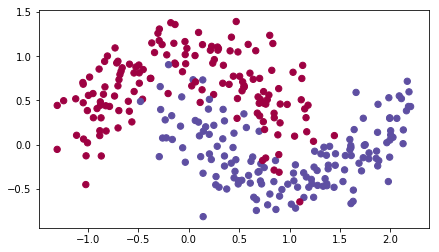We have already implemented a 3-layer neural network. You will train it with:

• update_parameters_with_gd()
• Mini-batch Momentum: it will call your functions:
• initialize_velocity() and update_parameters_with_momentum()
• initialize_adam() and update_parameters_with_adam()
In :
def model(X, Y, layers_dims, optimizer, learning_rate = 0.0007, mini_batch_size = 64, beta = 0.9,
beta1 = 0.9, beta2 = 0.999,  epsilon = 1e-8, num_epochs = 10000, print_cost = True):
"""
3-layer neural network model which can be run in different optimizer modes.

Arguments:
X -- input data, of shape (2, number of examples)
Y -- true "label" vector (1 for blue dot / 0 for red dot), of shape (1, number of examples)
layers_dims -- python list, containing the size of each layer
learning_rate -- the learning rate, scalar.
mini_batch_size -- the size of a mini batch
beta -- Momentum hyperparameter
beta1 -- Exponential decay hyperparameter for the past gradients estimates
beta2 -- Exponential decay hyperparameter for the past squared gradients estimates
num_epochs -- number of epochs
print_cost -- True to print the cost every 1000 epochs

Returns:
parameters -- python dictionary containing your updated parameters
"""

L = len(layers_dims)             # number of layers in the neural networks
costs = []                       # to keep track of the cost
t = 0                            # initializing the counter required for Adam update
seed = 10                        # For grading purposes, so that your "random" minibatches are the same as ours

# Initialize parameters
parameters = initialize_parameters(layers_dims)

# Initialize the optimizer
if optimizer == "gd":
pass # no initialization required for gradient descent
elif optimizer == "momentum":
v = initialize_velocity(parameters)

# Optimization loop
for i in range(num_epochs):

# Define the random minibatches. We increment the seed to reshuffle differently the dataset after each epoch
seed = seed + 1
minibatches = random_mini_batches(X, Y, mini_batch_size, seed)

for minibatch in minibatches:

# Select a minibatch
(minibatch_X, minibatch_Y) = minibatch

# Forward propagation
a3, caches = forward_propagation(minibatch_X, parameters)

# Compute cost
cost = compute_cost(a3, minibatch_Y)

# Backward propagation

# Update parameters
if optimizer == "gd":
elif optimizer == "momentum":
parameters, v = update_parameters_with_momentum(parameters, grads, v, beta, learning_rate)
t = t + 1 # Adam counter
t, learning_rate, beta1, beta2,  epsilon)

# Print the cost every 1000 epoch
if print_cost and i % 1000 == 0:
print ("Cost after epoch %i: %f" %(i, cost))
if print_cost and i % 100 == 0:
costs.append(cost)

# plot the cost
plt.plot(costs)
plt.ylabel('cost')
plt.xlabel('epochs (per 100)')
plt.title("Learning rate = " + str(learning_rate))
plt.show()

return parameters


You will now run this 3 layer neural network with each of the 3 optimization methods.

### 5.1 - Mini-batch Gradient descent¶

Run the following code to see how the model does with mini-batch gradient descent.

In :
# train 3-layer model
layers_dims = [train_X.shape, 5, 2, 1]
parameters = model(train_X, train_Y, layers_dims, optimizer = "gd")

# Predict
predictions = predict(train_X, train_Y, parameters)

# Plot decision boundary
axes = plt.gca()
axes.set_xlim([-1.5,2.5])
axes.set_ylim([-1,1.5])
plot_decision_boundary(lambda x: predict_dec(parameters, x.T), train_X, train_Y)

Cost after epoch 0: 0.690736
Cost after epoch 1000: 0.685273
Cost after epoch 2000: 0.647072
Cost after epoch 3000: 0.619525
Cost after epoch 4000: 0.576584
Cost after epoch 5000: 0.607243
Cost after epoch 6000: 0.529403
Cost after epoch 7000: 0.460768
Cost after epoch 8000: 0.465586
Cost after epoch 9000: 0.464518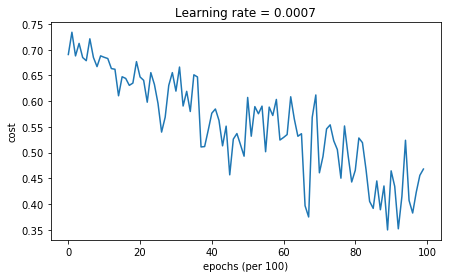Accuracy: 0.796666666667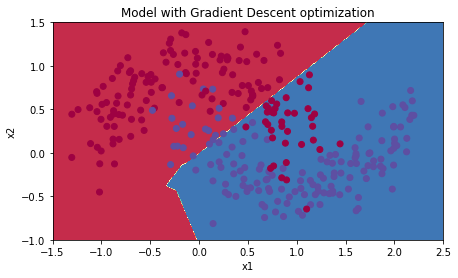### 5.2 - Mini-batch gradient descent with momentum¶

Run the following code to see how the model does with momentum. Because this example is relatively simple, the gains from using momemtum are small; but for more complex problems you might see bigger gains.

In :
# train 3-layer model
layers_dims = [train_X.shape, 5, 2, 1]
parameters = model(train_X, train_Y, layers_dims, beta = 0.9, optimizer = "momentum")

# Predict
predictions = predict(train_X, train_Y, parameters)

# Plot decision boundary
plt.title("Model with Momentum optimization")
axes = plt.gca()
axes.set_xlim([-1.5,2.5])
axes.set_ylim([-1,1.5])
plot_decision_boundary(lambda x: predict_dec(parameters, x.T), train_X, train_Y)

Cost after epoch 0: 0.690741
Cost after epoch 1000: 0.685341
Cost after epoch 2000: 0.647145
Cost after epoch 3000: 0.619594
Cost after epoch 4000: 0.576665
Cost after epoch 5000: 0.607324
Cost after epoch 6000: 0.529476
Cost after epoch 7000: 0.460936
Cost after epoch 8000: 0.465780
Cost after epoch 9000: 0.464740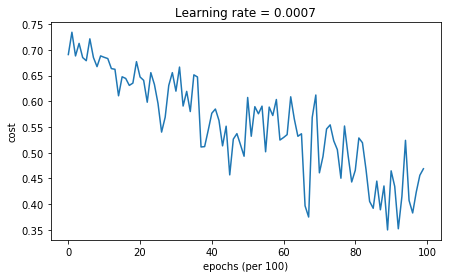Accuracy: 0.796666666667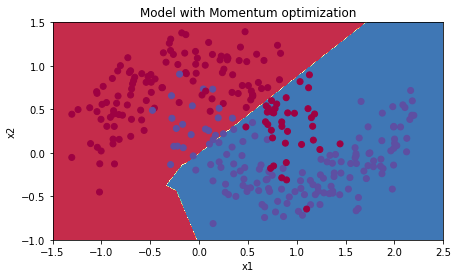### 5.3 - Mini-batch with Adam mode¶

Run the following code to see how the model does with Adam.

In :
# train 3-layer model
layers_dims = [train_X.shape, 5, 2, 1]
parameters = model(train_X, train_Y, layers_dims, optimizer = "adam")

# Predict
predictions = predict(train_X, train_Y, parameters)

# Plot decision boundary
axes = plt.gca()
axes.set_xlim([-1.5,2.5])
axes.set_ylim([-1,1.5])
plot_decision_boundary(lambda x: predict_dec(parameters, x.T), train_X, train_Y)

Cost after epoch 0: 0.690552
Cost after epoch 1000: 0.185501
Cost after epoch 2000: 0.150830
Cost after epoch 3000: 0.074454
Cost after epoch 4000: 0.125959
Cost after epoch 5000: 0.104344
Cost after epoch 6000: 0.100676
Cost after epoch 7000: 0.031652
Cost after epoch 8000: 0.111973
Cost after epoch 9000: 0.197940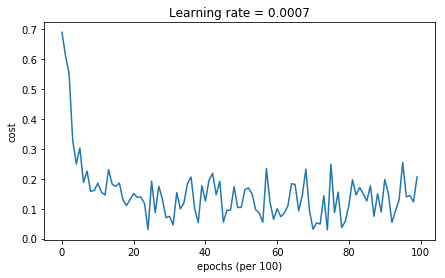Accuracy: 0.94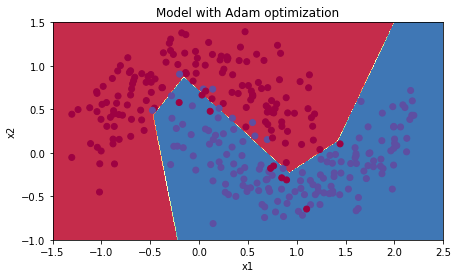### 5.4 - Summary¶

 **optimization method** **accuracy** **cost shape** Gradient descent 79.7% oscillations Momentum 79.7% oscillations Adam 94% smoother

Momentum usually helps, but given the small learning rate and the simplistic dataset, its impact is almost negligeable. Also, the huge oscillations you see in the cost come from the fact that some minibatches are more difficult thans others for the optimization algorithm.

Adam on the other hand, clearly outperforms mini-batch gradient descent and Momentum. If you run the model for more epochs on this simple dataset, all three methods will lead to very good results. However, you've seen that Adam converges a lot faster.

• Usually works well even with little tuning of hyperparameters (except $\alpha$)